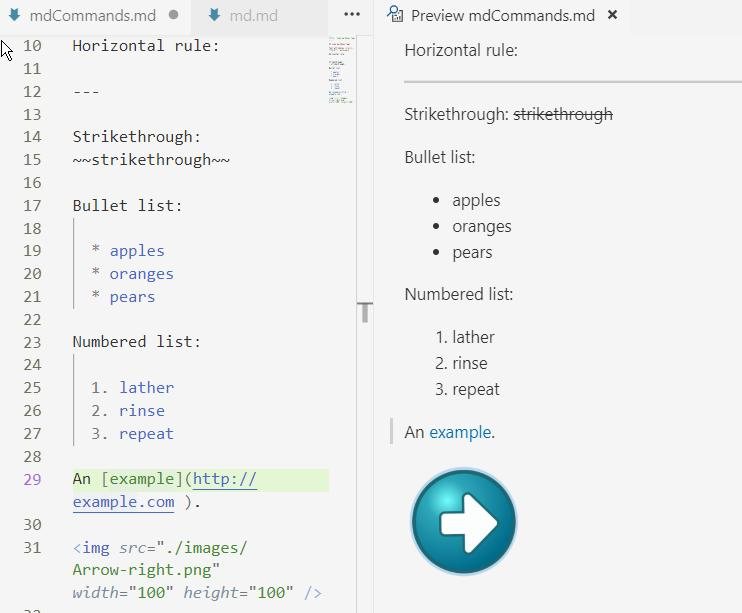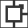# using markdown for documenting code

## introduction

Until now I have been writing my documentation in Wordpad, a simple text editor that is part of Windows since longtime. And if I write an article with a lot of mathematical equations I use Latex. Now I want to write some articles for the web, so my output must be a html-file. In the articles I will be using, apart from text, a little bit of math, some images and programming code. I am considering using markdown. The next figure shows some mardown on the left and a preview of the result on the left.## markdown

It is easy to write an article in the markdown format. The format uses markup symbols to make headings, to emphasize text, to insert hypertext links etc. The produced article is then saved as a normal text file with .md extension and that saved file can be used to produce the article in other formats like html (.html), open office (.odt), rich text format (.rtf). Markdown is used on all platforms (windows, linux, apple).

### editing

On all platforms there are editors for markup. Of course any text editor can be used, but these editors give extra support. I have three requirements. For me an essential support is to see the result of my typing live in a second window. A second requirement is to be able to insert programming code with syntax highlighting. As a final requirement I would like to be able to use markup for math.

The editor I'm using is Visual Studio Code (VS Code, not Visual Studio!, version 1.33). But there are other editors that will give similar support. Also there are online editors like Dillenger. VS Code is a free program. After starting VS Code look for a folder (menu File | Folder ...) in which the markdown will be created. After opening a .md file or creating a new file in VS Code the markup is shown in a window. Above that window is an icon to open a second window that shows the result of the markup (the window is also opened after Ctrl-K V).

### markdown syntax

The basic syntax of markdown was developed by John Gruber in 2004. The main concern of Gruber was the readability of the markup text (see: basic syntax). As an example of a tag, the hastag # is used to produce a heading. The heading of this paragraph was typed as ### markdown syntax.

Later new additions followed. For github, the site where many publish their programming efforts, a gfm (github favored markup) extension to the syntax was created that opens the possibility of producing code highlighting (see: gfm). The basic specification has some ambiguities, gfm is more precise. Code highlighting is an option in gfm. A code fence is used, starting with three backticks  (or three tildes ~~~) and ending with three backticks (or three tildes). Additionally an info string with the language of the code is added. As in the following example:

javascript
fq = function(x) {
var y = 12;
return 3 + y;
}


is rendered as:

fq = function(x) {
var y = 12;
return 3 + y;
}

Luckily, Visual Studio Code displays the gfm highlighting without problems (no new installations needed). As far as I know there is no markdown option to produce a line numbering for the code.

As far as math is concerned there are two options, either use Tex syntax for math or use Mathml syntax. Because typing MathMl is quite an effort, the only option really is Tex.

Math itself is not in markdown syntax available, but nothing prevents us from using an other syntax within markdown (well... almost nothing). To wite Tex/Latex we can use the conventional $signs. I did install the VS Code extension Markdown+Math (to install: click iconin the left menu bar and fill in the Search field Markdown+Math)). It seems VS Code uses Ka-tex. At the end of this file you can see that it works fine for inline math. It does not recognise double$\$ signs and also does not know about the 'equation' environment.

And now the remaining question is: will there be a processor/translator for the syntax we use. First of all, VS Code can convert the code to html after installing Markdown+Math. Press Ctrl+Shift+P to see the list of commands and fill the search bar with 'Markdown' and you will see that CTRK+K followed by ',' saves the markdown as html. But there are tools with more options. Look at what pandoc can do for us.

### pandoc processing

Pandoc is a processor that translates files from one format into another format. It is not the only program for this task, other programs are e.g. MultiMarkdown, Discount and Commonmark. It is a stand alone program that works from the command line. I'm using pandoc 2.9.1 that can be installed using an installer from github /pandoc.

After installing pandoc open a command prompt (in Windows 10 use the search button in the taskbar and enter Command). In de Command promt enter pandoc --help to view the available pandoc commands. Pandoc can translate all kinds of input formats (look at them in the Command Prompt, enter pandoc --list-input-formats) in all kinds of output formats (pandoc --list-output-formats). Markdown can be used as input as well as output, so can html, odt, mediawiki. An example of the simplest form for translation is pandoc mytext.md -f gfm -t html -s -o mytext.html, where a markdown file (in gfm style) is translated to a html file. But it is in the same way that a html file can be translated to a markdown file.

### templates

The html file produced by pandoc looks different from the preview you saw in VS Code. VS Code uses its own style. To change the default style of the pandoc translation use templates. For producing html we can use css templates. An example where a table of contents is produced and the template github-pandoc.css is used is the command: pandoc -s --toc -c github-pandoc.css -f gfm -t html md.md -o md3.html. Other examples are found here: demos on the pandoc site. The simplest way to 'integrate' the commands with VS Code is to make a batch file (extension .bat) that contains the translation command and to use that in a terminal of VS Code (choose menu Terminal | new Terminal) if a terminal is not already open.

Finally, lets look at math. Using javascript script libraries like Mathjax fairly wellformed math formula's can be produced. In the demos just mentioned examples are given for producing math in html. For Mathjax use: pandoc mymath.md -s --mathjax -o mathMathJax.html. The syntax of the math is Latex syntax and is put between single dollar signs for inline math, $$y=mx+c$$' is displayed as $$y=mx+c$$. For stand alone formulas the math is put between double dollar signs. Examples of the result can be seen in the next section.

To produce a pdf file the command is of course: pandoc mymath.md -s --mathjax -o mathMathJax.pdf. As a last step I did create a html file from this md.md file itself using the command pandoc -s --toc -c github-pandoc.css --mathjax md.md -f markdown-smart -t html -o md.html (note that I removed -f gfm from the command!) with a pleasing result, also for the math formulas in the next section! If you are now not looking at the source file md.md, you are looking at the result of this command md.html. Even the matrices are displayed as they should.

### Some final points

As can be seen from the pandoc --help command there are many more options. The options -A (After) and -B (Before) to include text before and after the body can come in quit handy. In the manual is told how pandoc works. It has a scripting language of its own. The script used for converting to html can be seen by giving the following command in the command prompt: 'pandoc -D html'. Pandoc also has its own extensions to markdown. For instance, it supports footnotes, like this: Text with a footnote 1 (also available in VS Code after downloading the extension 'Markdown Footnotes').

Of course, much more can be said on markdown. There are also markdown machines that give the option of inserting executable code (see Maruku and R Markdown). Using these options will convert your markdown in some kind of workbook environment! For now, I am satisfied with the possibilities offered by VS code, the syntax supported by pandoc and the processing of Pandoc.

# math test section

An extra section for testing math in a markdown file. The formula, $$y=mx+c$$, is displayed inline. Some symbols and equations (such as $$\sum{x}$$ or $$\frac{1}{2}$$) are rescaled to prevent disruptions to the regular line spacing. For more voluminous equations (such as $$\sum{\frac{(\mu - \bar{x})^2}{n-1}}$$), some line spacing disruptions are unavoidable.
Math should then be displayed in displayed mode. $\sum{\frac{(\mu - \bar{x})^2}{n-1}}$

$\begin{equation*} A = \begin{pmatrix} 1 & 2 & 3 \\ 4 & 50 & 60 \\ 7 & 8 & 90 \end{pmatrix} \quad \begin{pmatrix} 10 & 20 & 30 \\ 40 & 5 & 6 \\ 70 & 80 & 9 \end{pmatrix} \end{equation*}$